Type Here to Get Search Results !

# MATHEMATICS FORM FOUR TOPIC 8: LINEAR PROGRAMMING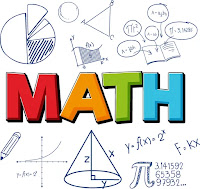LINEAR PROGRAMMING
Simultaneous Equation from Word Problems
Form simultaneous equation from word problems
LINEAR PROGRAMMING.
Linear programming - is a branch of mathematics which deals with either minimizing the cost or maximizing the profit.
• It gives the best way of utilizing the scarce resources available.
• It is so called because it only involves equations and inequalities which are linear.
Simultaneous Equation.
One of the methods used in solving linear simultaneous equations is a graphical method. Two linear simultaneous equations in two unknowns can be graphically solved by passing through the following procedures.
1. Draw the two lines which represent the two equations on the xy – plane this is done by deter mining at least two points through which each line passes, the intercept are commonly used
2. Determine the point of intersection of the two lines. This point of intersection is the solution to the system of equations.
FACT:
• If two straight lines are not parallel then they meet at only one point:
• In case the lines do not meet, there is no solution to the corresponding system of simultaneous equations.
Example 1
Graphically solve the following system of simultaneous equations.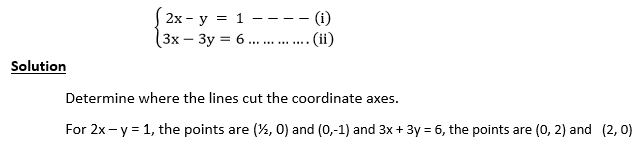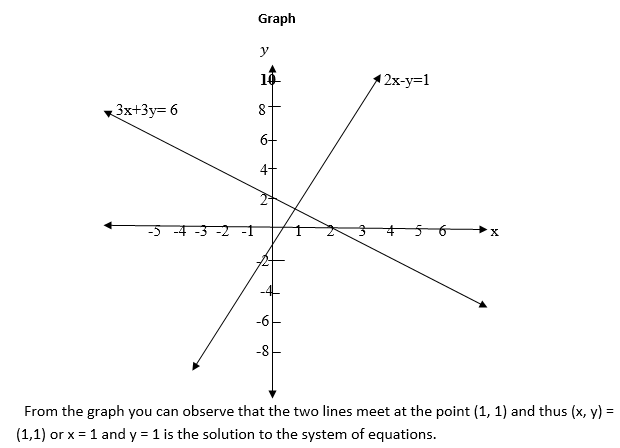Example 2
Find the solution to the following system of simultaneous equations by graphical method.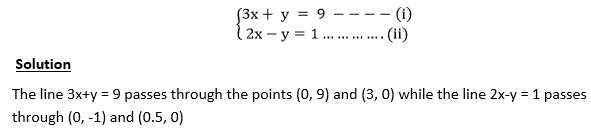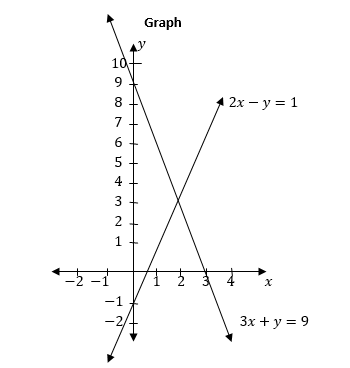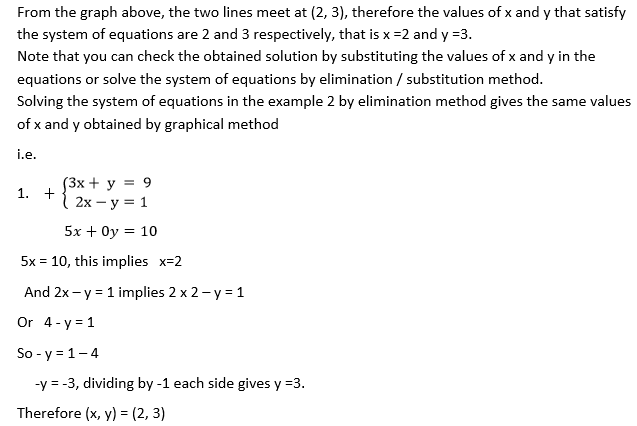Solving Simultaneous Equations Graphically
Solve simultaneous equations graphically
Example 3
Solve the following simultaneous equations graphically and check your solution by a non-graphical method: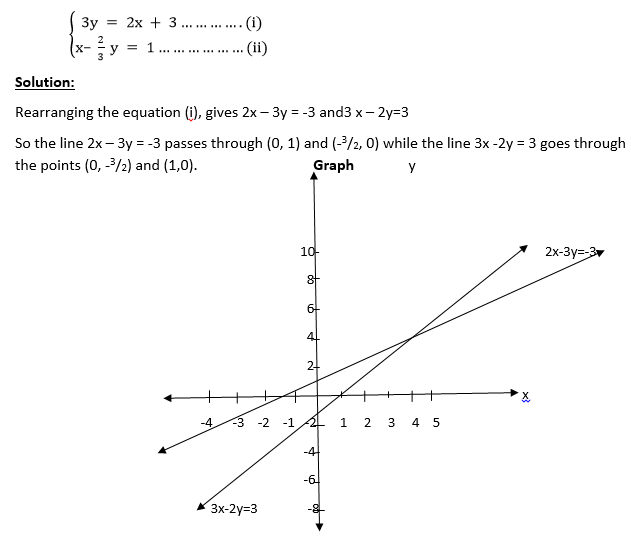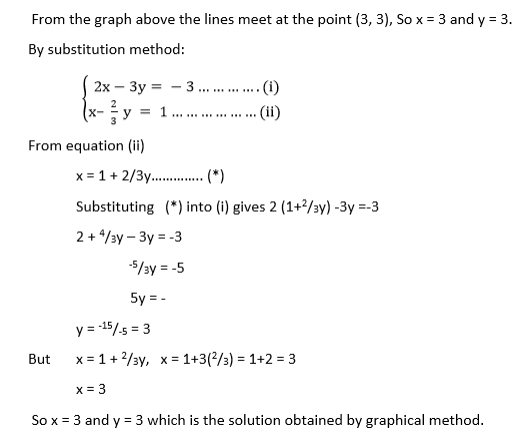Example 4
Find the solution to the following system of simultaneous equations by graphical method.Exercise 1
Find the solution to the following systems of simultaneous equations graphically.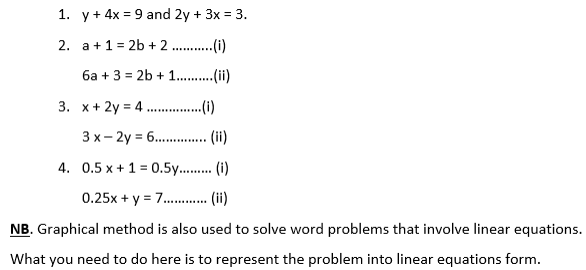Try: Ali paid 34 shillings for 10 oranges and 35 mangoes. Moshi went to the same market and paid 24 shillings for 16 oranges and 18 mangoes. What was the price for a mango and for an orange?

Forming Linear Inequalities in Two Unknowns from Word Problems
Form linear inequalities in two unknowns from word problems
Linear inequalities
• Normally any straight line drawn on xy – plane separates it into two disjoint sets. These sets are called half – planes
• Consider the equation y = 5 drawn on the xy plane as shown below.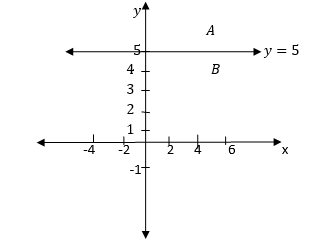From the figure above, all points above the line, that is all points in the half plane A which is above the line satisfy the relation y>5 and those lying in the half plane B which is below the given line, satisfy the relation y< 5.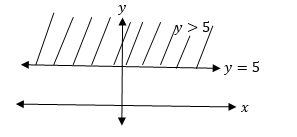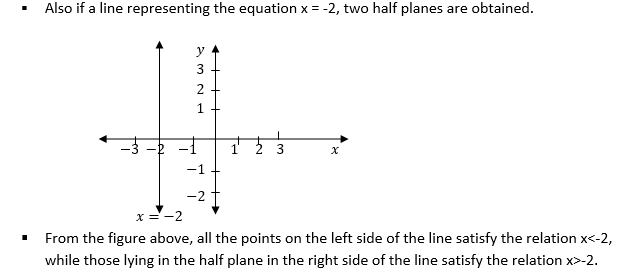• In linear programming usually the region of interest is left clear that is we shade unwanted region(s).
NB:
When shading the half planes we consider the inequalities as the equations but dotted lines are used for the relations with > or < signs and normal lines are used for those with ≥ or ≤ signs.
Consider the inequalities x>0, y>0 and 2x + 3y >12 represented on the xy-plane In this case we draw the line x=0, y= 0 and 2x+3y=12 but the point about the inequality signs for each equation must be considered.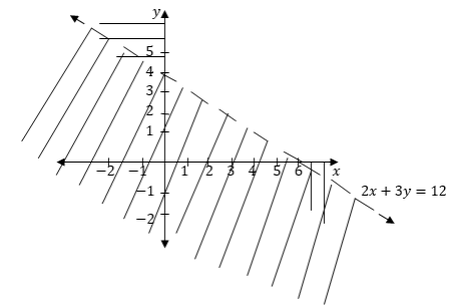From the figure above, the clear region satisfy all the inequalitiesx>0, y>0 and 2x + 3y >12, these three lines are the boundaries of the region.
The Solution Set of Simultaneous Linear Inequalities Graphically
Find the solution set of simultaneous linear inequalities graphically
Example 5
Draw and show the half plane represented by 8x + 2y ≥16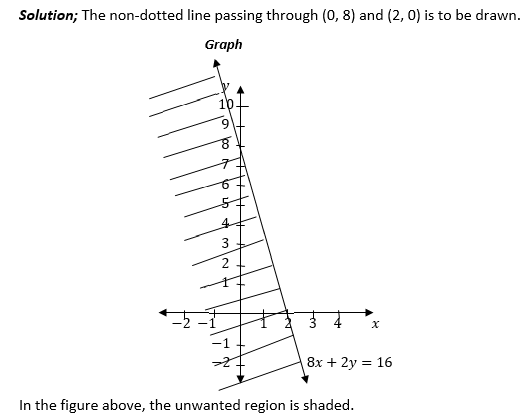Feasible Region
Definition: In the xy plane the region that satisfies all the given inequalities is called the feasible region (F.R)
Example 6
Indicate the feasible region for the inequalities 2x+3y ≥ 12 and y-x ≤ 2.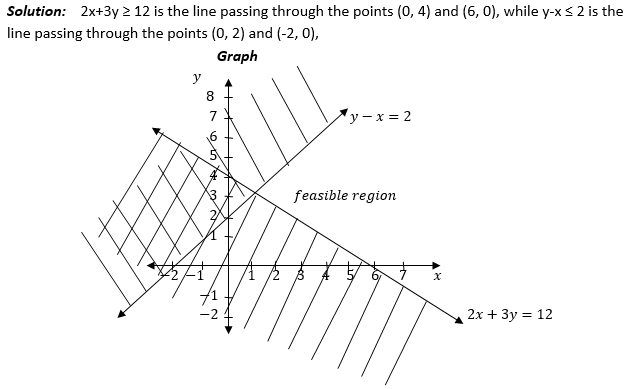Determine the solution set of the simultaneous inequalities y + x ≥3 and x-2y ≤ 9.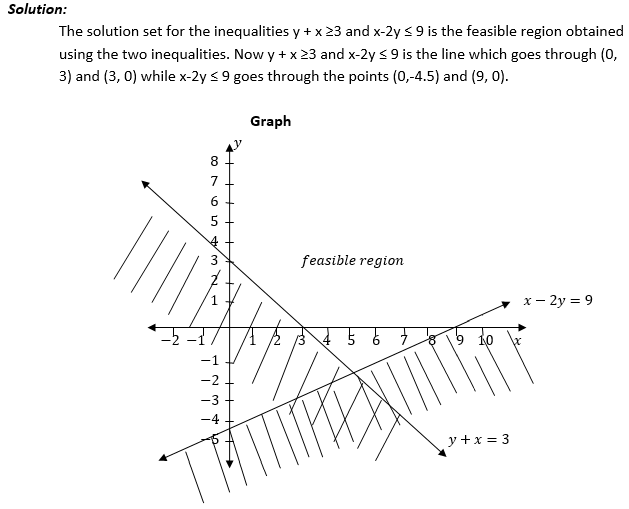Example 7
Fatuma was given 30 shillings to buy oranges and mangoes. An orange costs 2shillings while a mango costs 3 shillings. If the number of oranges bought is at least twice the number of mangoes, show graphically the feasible region representing the number of ranges and mangoes she bought, assuming that no fraction of oranges and mangoes are sold at the market.
Solution:-
Le x be the number of oranges she bought and y the number of mangoes she bought. Now the cost of x and y together is 2x + 3y shillings which must not exceed 30 shillings. Inequalities:
2x + 3y ≤30 ……… (i) and x≥2y …………….. (ii),
Also because there is no negative oranges or mangoes that can be bought,
then x≥ and y≥0 ……….. (iii)
Now the line 2x + 3y ≤30 is the line passing through (0, 10) and (15,0) and the line x≥2y or x – 2y ≥ 0 is the line which passes through (0,0) and (2,1).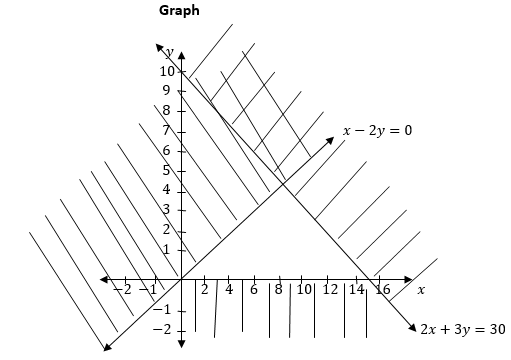Exercise 2
For practice.
1. Draw the graph of the equation 2x – y = 7 and show which half plane is represented by 2x – y >7 and the one represented by 2x – y <7 li="">
2. On the same coordinate axes draw the graphs of the following inequalities: x + 2y ≤ 2, y-x ≤ 1 and y ≥ 0.
3. Draw the graphs of y < 2x -1 and y > 3 – x on the same axes and indicate the feasible region.
4. A post office has to transport 870 parcels using a lorry, which takes 150 parcels at a time and a van which can take 60 at a time. The cost of each journey is 350 shillings by lorry and 280 shillings by van. The van makes more trips than the lorry and the total cost should not exceed 3080 shillings. Show graphically the feasible region representing the number of trips that a lorry and a van can make.

An Objective Function from Word Problems
Form an objective function from word problems
Linear programming components
Any linear programming problem has the following:
1. Objective
2. Alternative course (s) of action which will achieve the objective.
3. The available resources which are in limited supply.
4. The objective and its limitations should be able to be expressed as either linear mathematical equations or linear inequalities. Therefore linear programming aims at finding the best use of the available resources.
Programmingis the use of mathematical techniques in order to get the best possible solution to the problem
Steps to be followed in solving linear programming problems;
1. Read carefully the problem, if possible do it several times.
2. Use the variables like x and y to represent the resources of interest.
3. Summarize the problem by putting it in mathematical form using the variables let in step (b) above. In this step you need to formulate the objective function and inequalities or constraints.
4. Plot the constraints on a graph
5. From your graph, identify the corner points.
6. Use the objective function to test each corner point to find out which one gives the optimum solution.
7. Make conclusion after finding or identifying the optimum point among the corner points.
Corner Points on the Feasible Region
Locate corner points on the feasible region
Example 8
A student has 1200 shillings to spend on exercise books. At the school shop an exercise book costs 80shillings, and at a stationery store it costs 120 shillings. The school shop has only 6 exercise books left and the student wants to obtain the greatest number of exercise books possible using the money he has. How many exercise books will the student buy from each site?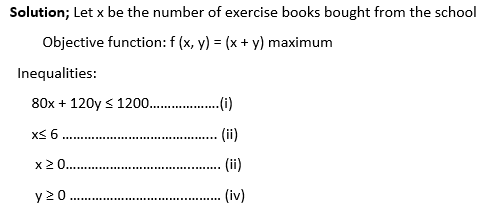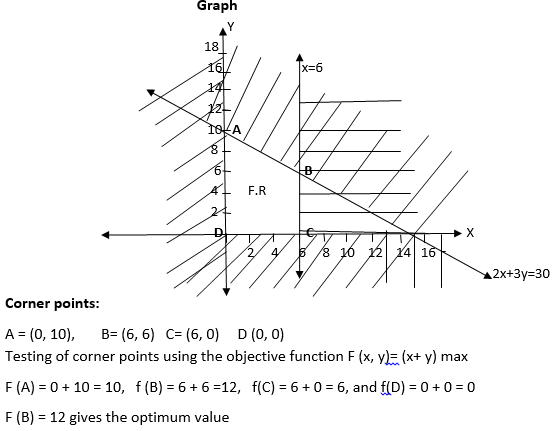Therefore the student will buy 6 exercise books from each site.
Example 9
A nutritionist prescribes a special diet for patients containing the following number of Units of vitamins A and B per kg, of two types of food f1 and f2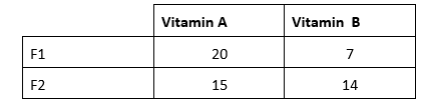If the daily minimum in take required is 120 Units of A and 70 units of B, what is the least total mass of food a patient must have so as to have enough of these vitamins?
Solution:
Let x be the number of kg(s) of F1 that patient gets daily and y be the number of kg(s) of Fto be taken by the patient daily.
Objective function: F (x, y) = (x + y) minimum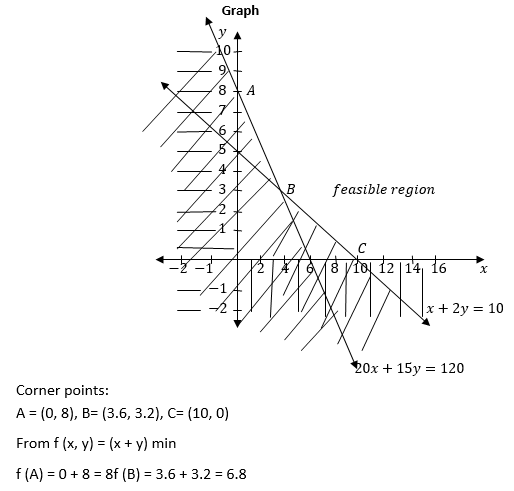f (C) = 10 + 0 = 10
So f (B) = 6.8 is the minimum
Therefore the least total mass of food the patient must have is 6.8 kilograms
The Minimum and Maximum Values using the Objective Functio
Find the minimum and maximum values using the objective function
Example 10
A farmer wants to plant coffee and potatoes. Coffee needs 3 men per hectare while potatoes need also 3 men per hectare. He has 48 hired laborers available. To maintain a hectare of coffee he needs 250 shillings while a hectare of potatoes costs him 100 shillings. .
Find the greatest possible land he can sow if he is prepared to use 25,000 shillings.
Solution:
Let x be the number of hectares of coffee to be planted and y be the number of hectares of potatoes to be planted.
Objective function: f (x, y) = (x, + y) maximum
3x + 3y ≤ 48 or x + y ≤16 ………….(i)
250x + 100y≤ 25,000 Or 5x + 2y ≤ 500………(ii)
x ≥ 0 …………………...(iii)
y≥ 0 …………………...(iv)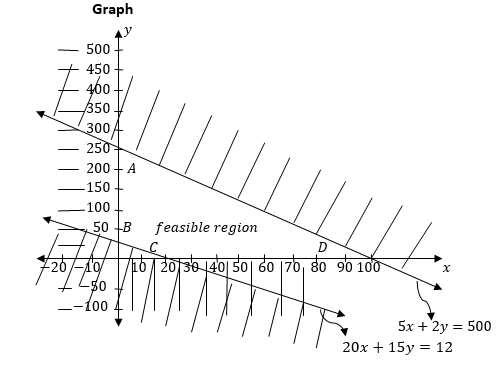Using the objective function f (x, y) = (x + y) maximum,
f (A) = (0 + 250) = 250
f (B) = (0+16) = 16
f (C) = (16+0) = 16
f(D) = (100+0)= 100 (maximum)
Therefore the greatest possible area to be planted is 250 hectors of potatoes.
NB: In most cases L.P problems must involve non-negativity constraints (inequalities) that are x ≥ 0 and y ≥ 0. This is due to the fact that in daily practice there is no use of negative quantities.
Example 11
A technical school is planning to buy two types of machines. A lather machine needs 3m2 of floor space and a drill machine needs 2m2 of floor space. The total space available is 30m2. The cost of one lather machine is 25,000 shillings and that of drill machine is 30,000 shillings. The school can spend not more than 300,000 shillings, what is the greatest number of machines the school can buy?
Solution:
Let x be the number of Lather machines and y be the number of drill machines to be bought
Objective function: f(x, y) = (x + y) max
Inequalities:
3x + 2y ≤ 30.. ………………….(i)
25,000x + 30,000y ≤300,000
Or 5x + 6y ≤ 60……………………..(ii)
x ≥ 0 ……………………………….(iii)
y ≥ 0…………………… ………….(iv)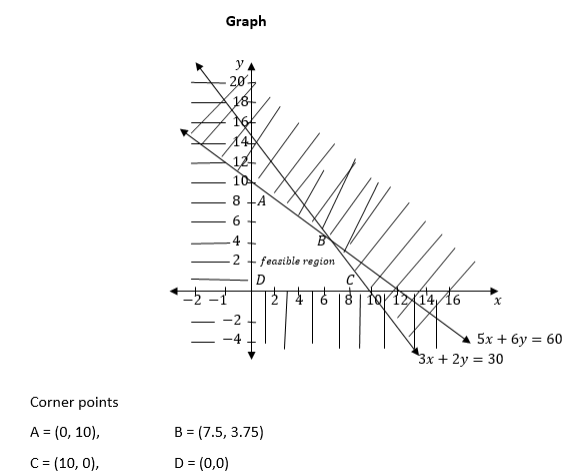Since the incomplete machine can’t work, then B = (8, 3) or (7, 4).That is approximating values of x and y to the possible integers without affecting the given inequalities or conditions.
Now by using the objective function,
f (A) = 0 + 10 = 10
f(B) = 7 + 4 0r f (B) = 8 + 3 = 11
f (C) = 10 + 0 = 10
f (D) = 0 + ) = 0
So f (B) gives the maximum number of machines which is 11.
Therefore the greatest number of machines that can be bought by the school is 11 machines.
Exercise 3
1. Show on a graph the feasible region for which the restrictions are:
y ≤ 2x, x≥ 6, y≥2 and 2x + 3y ≤30
From the graph at which point does:
1. y – x take a maximum value?
2. x + y take a maximum value?
3. y – x take a maximum value?
2. With only 20,000 shillings to spend on fish, John had the choice of buying two types of fish. The price of a single fish type 1 was 2,500shillings and each fish of type 2 was sold at 2,000 shillings. He wanted to buy at least four of type 1. What is the greatest number of fish did John buy? How many of each type could he buy?
3. How many corner points does the feasible region restricted by the inequalities?
x≥0, y ≥ 0, 3x + 2y ≤ 18 and 2x + 4y ≤16 have?
Which corner point maximizes the objective function f (x, y) = 2x + 5y?

Tags